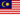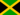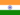All Modules View all topics Close topics
Introduction to electric systems

Voltage

• Study Notes
• Discuss This TopicQayyum M. 0 0 Can someone clarify me on this, please? "A light globe that is causing a voltage drop of 20 volts may actually mean that a coulomb of charge with 240 joules of energy passes through the globe and exits with only 220 joules of energy." Thanks in advance :)Sheneil C. 0 0 So voltage is energy/CoulombManish C. 0 0 The term voltage refers to the amount of energy given to a coulomb of charge as it passes through a battery or supply, or the amount of energy that a coulomb of charge gives up as it passes through a load. The term voltage refers to an energy change, also called a potential difference; actual energy values are not important. For example, a nine volt battery gives each passing coulomb nine more joules of energy regardless of the energy that the coulomb of charge may already possess. A light globe that is causing a voltage drop of 20 volts may actually mean that a coulomb of charge with 240 joules of energy passes through the globe and exits with only 220 joules of energy. When measuring a voltage value, the voltmeter is essentially comparing energy values either side of a circuit component, hence a voltmeter is placed 'in parallel' in a circuit.Anudeep S. 1 0 I couldn't get the meaning of globe. Can someone help?
• Text Version